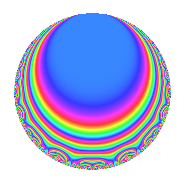Properties

 Label 6012.2.a.eLevel 6012 Weight 2 Character orbit 6012.a Self dual Yes Analytic conductor 48.006 Analytic rank 1 Dimension 5 CM No Inner twists 1

Related objects

Newspace parameters

 Level: $$N$$ = $$6012 = 2^{2} \cdot 3^{2} \cdot 167$$ Weight: $$k$$ = $$2$$ Character orbit: $$[\chi]$$ = 6012.a (trivial)

Newform invariants

 Self dual: Yes Analytic conductor: $$48.0060616952$$ Analytic rank: $$1$$ Dimension: $$5$$ Coefficient field: 5.5.149169.1 Coefficient ring: $$\Z[a_1, \ldots, a_{7}]$$ Coefficient ring index: $$1$$ Fricke sign: $$1$$ Sato-Tate group: $\mathrm{SU}(2)$

$q$-expansion

Coefficients of the $$q$$-expansion are expressed in terms of a basis $$1,\beta_1,\beta_2,\beta_3,\beta_4$$ for the coefficient ring described below. We also show the integral $$q$$-expansion of the trace form.

 $$f(q)$$ $$=$$ $$q$$ $$+ ( \beta_{1} - \beta_{3} + \beta_{4} ) q^{5}$$ $$+ ( -\beta_{1} - \beta_{4} ) q^{7}$$ $$+O(q^{10})$$ $$q$$ $$+ ( \beta_{1} - \beta_{3} + \beta_{4} ) q^{5}$$ $$+ ( -\beta_{1} - \beta_{4} ) q^{7}$$ $$+ ( -\beta_{1} + \beta_{3} - \beta_{4} ) q^{11}$$ $$+ ( -1 + \beta_{1} - \beta_{2} - \beta_{3} + \beta_{4} ) q^{13}$$ $$+ ( 3 - 2 \beta_{1} - 2 \beta_{2} - 2 \beta_{4} ) q^{17}$$ $$+ ( -1 + 2 \beta_{1} + \beta_{2} + \beta_{3} + \beta_{4} ) q^{19}$$ $$+ ( -3 + \beta_{1} + 2 \beta_{2} - \beta_{4} ) q^{23}$$ $$+ ( -1 + \beta_{1} - \beta_{2} - \beta_{3} + 2 \beta_{4} ) q^{25}$$ $$+ ( -\beta_{1} + \beta_{2} + 3 \beta_{3} + 2 \beta_{4} ) q^{29}$$ $$+ ( -3 + 2 \beta_{2} + \beta_{3} ) q^{31}$$ $$+ ( -1 - \beta_{1} + \beta_{3} - 2 \beta_{4} ) q^{35}$$ $$+ ( -4 - \beta_{1} + 4 \beta_{2} - \beta_{3} + 2 \beta_{4} ) q^{37}$$ $$+ ( 2 - \beta_{1} + 4 \beta_{2} + 2 \beta_{3} ) q^{41}$$ $$+ ( 2 + \beta_{1} - 3 \beta_{2} + 2 \beta_{3} - \beta_{4} ) q^{43}$$ $$+ ( -4 \beta_{1} - \beta_{2} + \beta_{3} + \beta_{4} ) q^{47}$$ $$+ ( -4 + \beta_{2} - \beta_{3} ) q^{49}$$ $$+ ( -1 + 3 \beta_{1} - \beta_{2} + 2 \beta_{4} ) q^{53}$$ $$+ ( -4 - \beta_{1} + \beta_{2} + \beta_{3} - 2 \beta_{4} ) q^{55}$$ $$+ ( 1 + 3 \beta_{1} - \beta_{2} - 2 \beta_{4} ) q^{59}$$ $$+ ( -4 - 4 \beta_{1} - \beta_{2} + 2 \beta_{3} + \beta_{4} ) q^{61}$$ $$+ ( 5 + \beta_{1} - 3 \beta_{2} - \beta_{3} ) q^{65}$$ $$+ ( 3 - \beta_{1} - \beta_{2} + \beta_{3} - 5 \beta_{4} ) q^{67}$$ $$+ ( -5 + 4 \beta_{1} + 3 \beta_{2} - 2 \beta_{3} + 4 \beta_{4} ) q^{71}$$ $$+ ( -4 + 4 \beta_{1} - 4 \beta_{2} - 2 \beta_{3} - \beta_{4} ) q^{73}$$ $$+ ( 1 + \beta_{1} - \beta_{3} + 2 \beta_{4} ) q^{77}$$ $$+ ( -6 - 5 \beta_{1} + \beta_{2} + 2 \beta_{3} + \beta_{4} ) q^{79}$$ $$+ ( 1 + 4 \beta_{1} - 2 \beta_{2} - 2 \beta_{3} - 5 \beta_{4} ) q^{83}$$ $$+ ( 3 \beta_{1} - 4 \beta_{2} - 3 \beta_{3} - 3 \beta_{4} ) q^{85}$$ $$+ ( 7 - 2 \beta_{1} - 3 \beta_{2} - 3 \beta_{3} - \beta_{4} ) q^{89}$$ $$+ ( -3 + \beta_{1} + \beta_{2} + 2 \beta_{3} + \beta_{4} ) q^{91}$$ $$+ ( -2 - 2 \beta_{1} + 2 \beta_{2} + \beta_{3} + 2 \beta_{4} ) q^{95}$$ $$+ ( -4 - 2 \beta_{2} + 3 \beta_{3} - 3 \beta_{4} ) q^{97}$$ $$+O(q^{100})$$ $$\operatorname{Tr}(f)(q)$$ $$=$$ $$5q$$ $$\mathstrut +\mathstrut 3q^{5}$$ $$\mathstrut -\mathstrut 2q^{7}$$ $$\mathstrut +\mathstrut O(q^{10})$$ $$5q$$ $$\mathstrut +\mathstrut 3q^{5}$$ $$\mathstrut -\mathstrut 2q^{7}$$ $$\mathstrut -\mathstrut 3q^{11}$$ $$\mathstrut -\mathstrut 4q^{13}$$ $$\mathstrut +\mathstrut 7q^{17}$$ $$\mathstrut -\mathstrut 2q^{19}$$ $$\mathstrut -\mathstrut 13q^{23}$$ $$\mathstrut -\mathstrut 2q^{25}$$ $$\mathstrut +\mathstrut 3q^{29}$$ $$\mathstrut -\mathstrut 12q^{31}$$ $$\mathstrut -\mathstrut 10q^{35}$$ $$\mathstrut -\mathstrut 7q^{37}$$ $$\mathstrut +\mathstrut 16q^{41}$$ $$\mathstrut -\mathstrut q^{47}$$ $$\mathstrut -\mathstrut 17q^{49}$$ $$\mathstrut -\mathstrut 3q^{53}$$ $$\mathstrut -\mathstrut 23q^{55}$$ $$\mathstrut -\mathstrut q^{59}$$ $$\mathstrut -\mathstrut 22q^{61}$$ $$\mathstrut +\mathstrut 20q^{65}$$ $$\mathstrut +\mathstrut 2q^{67}$$ $$\mathstrut -\mathstrut 9q^{71}$$ $$\mathstrut -\mathstrut 28q^{73}$$ $$\mathstrut +\mathstrut 10q^{77}$$ $$\mathstrut -\mathstrut 28q^{79}$$ $$\mathstrut -\mathstrut 7q^{83}$$ $$\mathstrut -\mathstrut 11q^{85}$$ $$\mathstrut +\mathstrut 30q^{89}$$ $$\mathstrut -\mathstrut 13q^{91}$$ $$\mathstrut -\mathstrut 3q^{95}$$ $$\mathstrut -\mathstrut 33q^{97}$$ $$\mathstrut +\mathstrut O(q^{100})$$

Basis of coefficient ring in terms of a root $$\nu$$ of $$x^{5}\mathstrut -\mathstrut$$ $$6$$ $$x^{3}\mathstrut -\mathstrut$$ $$3$$ $$x^{2}\mathstrut +\mathstrut$$ $$4$$ $$x\mathstrut +\mathstrut$$ $$1$$:

 $$\beta_{0}$$ $$=$$ $$1$$ $$\beta_{1}$$ $$=$$ $$\nu$$ $$\beta_{2}$$ $$=$$ $$\nu^{3} - \nu^{2} - 4 \nu + 1$$ $$\beta_{3}$$ $$=$$ $$\nu^{4} - \nu^{3} - 4 \nu^{2} + \nu$$ $$\beta_{4}$$ $$=$$ $$\nu^{4} - \nu^{3} - 5 \nu^{2} + \nu + 3$$
 $$1$$ $$=$$ $$\beta_0$$ $$\nu$$ $$=$$ $$\beta_{1}$$ $$\nu^{2}$$ $$=$$ $$-$$$$\beta_{4}\mathstrut +\mathstrut$$ $$\beta_{3}\mathstrut +\mathstrut$$ $$3$$ $$\nu^{3}$$ $$=$$ $$-$$$$\beta_{4}\mathstrut +\mathstrut$$ $$\beta_{3}\mathstrut +\mathstrut$$ $$\beta_{2}\mathstrut +\mathstrut$$ $$4$$ $$\beta_{1}\mathstrut +\mathstrut$$ $$2$$ $$\nu^{4}$$ $$=$$ $$-$$$$5$$ $$\beta_{4}\mathstrut +\mathstrut$$ $$6$$ $$\beta_{3}\mathstrut +\mathstrut$$ $$\beta_{2}\mathstrut +\mathstrut$$ $$3$$ $$\beta_{1}\mathstrut +\mathstrut$$ $$14$$

Embeddings

For each embedding $$\iota_m$$ of the coefficient field, the values $$\iota_m(a_n)$$ are shown below.

For more information on an embedded modular form you can click on its label.

Label $$\iota_m(\nu)$$ $$a_{2}$$ $$a_{3}$$ $$a_{4}$$ $$a_{5}$$ $$a_{6}$$ $$a_{7}$$ $$a_{8}$$ $$a_{9}$$ $$a_{10}$$
1.1
 −1.81853 2.54970 −1.25464 −0.228573 0.752046
0 0 0 −2.12560 0 0.221696 0 0 0
1.2 0 0 0 −0.951283 0 −1.28171 0 0 0
1.3 0 0 0 0.171230 0 2.92708 0 0 0
1.4 0 0 0 2.71918 0 −2.29630 0 0 0
1.5 0 0 0 3.18647 0 −1.57076 0 0 0
 $$n$$: e.g. 2-40 or 990-1000 Embeddings: e.g. 1-3 or 1.5 Significant digits: Format: Complex embeddings Normalized embeddings Satake parameters Satake angles

Inner twists

This newform does not admit any (nontrivial) inner twists.

Atkin-Lehner signs

$$p$$ Sign
$$2$$ $$-1$$
$$3$$ $$-1$$
$$167$$ $$1$$

Hecke kernels

This newform can be constructed as the kernel of the linear operator $$T_{5}^{5}$$ $$\mathstrut -\mathstrut 3 T_{5}^{4}$$ $$\mathstrut -\mathstrut 7 T_{5}^{3}$$ $$\mathstrut +\mathstrut 16 T_{5}^{2}$$ $$\mathstrut +\mathstrut 15 T_{5}$$ $$\mathstrut -\mathstrut 3$$ acting on $$S_{2}^{\mathrm{new}}(\Gamma_0(6012))$$.